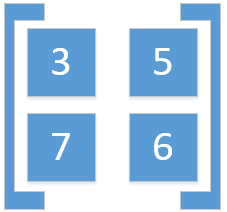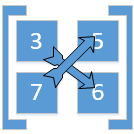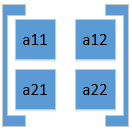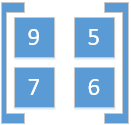# Determinants

##### 368

Every square matrix A = [aij] of order n, can be associate to a number. Which can be real or complex. Such Number is called as a Determinant.

eg , given the following matrix A of order 2,Order of the following matrix will be:Det of A = 3*5 - 7*5

Det A=-20

### Determinant of Matrix of Order 1

Given the the matrix A of order 1 =Since there's only one element in it, hence the DET of A = 3.

### Determinant of Matrix of Order 2

Given the following matrix of Order 2:

A =DET of A = ( a11 * a22 ) - ( a12 * a21)

eg if Matrix B =DET of B = ( 9*6 ) - ( 5*7 ) =

=    54-35 = 19